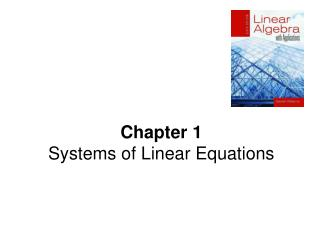# Chapter 1 Systems of Linear Equations - PowerPoint PPT PresentationDownload PresentationChapter 1 Systems of Linear Equations

Presentation Description
Download Presentation## Chapter 1 Systems of Linear Equations

- - - - - - - - - - - - - - - - - - - - - - - - - - - E N D - - - - - - - - - - - - - - - - - - - - - - - - - - -
##### Presentation Transcript

1. Chapter 1Systems of Linear Equations

2. A B i.e., Summary Use row operations to [A: B] : Def. [In: X] is called the reduced echelon form of [A : B]. Note.1. If A is the matrix of coefficients of a system of n equations in n variables that has a unique solution, then A is row equivalent toIn (AIn). 2. If AIn, then the system has unique solution.

3. R2+(–2)R1 R3+R1 Example 4 Many Systems Solving the following three systems of linear equation, all of which have the same matrix of coefficients. Solution The solutions to the three systems are

4. 1-2 Gauss-Jordan Elimination • Definition • A matrix is in reduced echelon form if • Any rows consisting entirely of zeros are grouped at the bottom of the matrix. • The first nonzero element of each other row is 1. This element is called a leading 1. • The leading 1 of each row after the first is positioned to the right of the leading 1 of the previous row. • All other elements in a column that contains a leading 1 are zero.

5. Examples for reduced echelon form () () () () • elementary row operations，reduced echelon form • The reduced echelon form of a matrix is unique.

6. Gauss-Jordan Elimination • System of linear equations  augmented matrix  reduced echelon form solution

7. pivot (leading 1) pivot Example 1 Use the method of Gauss-Jordan elimination to find reduced echelon form of the following matrix. Solution The matrix is the reduced echelon form of the given matrix.

8. Example 2 Solve, if possible, the system of equations Solution The general solution to the system is

9. Example 3 This example illustrates a system that has no solution. Let us try to solve the system Solution 0x1+0x2+0x3=1 The system has no solution.

10. Example: Theorem 1.1 A system of homogeneous linear equations in n variables always has the solution x1 = 0, x2 = 0. …, xn = 0. This solution is called the trivial solution. Observe that is a solution. Homogeneous System of linear Equations Definition Asystem of linear equations is said to be homogeneous if all the constant terms are zeros.

11. Example: The system has other nontrivial solutions. Theorem 1.2 A system of homogeneous linear equations that has more variables than equations has many solutions. Homogeneous System of linear Equations Note. Non trivial solution